# JAC Class 10 Maths Solutions Chapter 13 Surface Areas and Volumes Ex 13.1

Jharkhand Board JAC Class 10 Maths Solutions Chapter 13 Surface Areas and Volumes Ex 13.1 Textbook Exercise Questions and Answers.

## JAC Board Class 10 Maths Solutions Chapter 13 Surface Areas and Volumes Exercise 13.1

Unless stated otherwise, take π = $$\frac{22}{7}$$

Question 1.
Two cubes each of volume 64 cm3 are joined end to end. Find the surface area of the resulting cuboid.
Solution:
Volume of the cube = a3 = 64 cm3
Side of the cube $$\sqrt{64}$$ = a = 4 cm.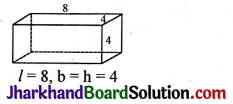Surface area of the cuboid = 2(lb + bh + lh)
= 2[(8 × 4) + (4 × 4) + (8 × 4)]
= 2[32 + 16 + 32]
= 2 × 80
= 160 cm2.

Question 2.
A vessel is in the form of a hollow hemisphere mounted by a hollow cylinder. The diameter of the hemisphere is 14 cm and the total height of the vessel is 13 cm. Find the inner surface area of the vessel.
Solution: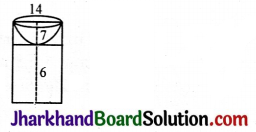π = $$\frac{22}{7}$$, radius of the hemisphere = 7 cm,
height of the hemisphere = 7 cm,
height of the cylinder, h = 13 – 7 = 6 cm.
Inner area of the vessel = Inner area of the hemisphere vessel + Inner area of the cylinder
= 2πr2 + 2πrh
= 2 × $$\frac{22}{7}$$ × 7 × 7 + 2 × $$\frac{22}{7}$$ × 7 × 6
= 2 × 22 × 7+2 × 22 × 6
= 2 × 22(7 + 6)
= 44 × 13 = 572 cm2.Question 3.
A toy is in the form of a cone of radius 3.5 cm mounted on a hemisphere of same radius. The total height of the toy is 15.5 cm. Find the total surface area of the toy.
Solution: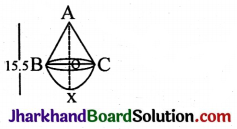π = $$\frac{22}{7}$$, r = 3.5, l = ?
Total surface area of the toy = C.S.A. of the cone + C.S.A. of the hemisphere
= πrl + 2πr2
lv = r2 + h2
= (3.5)2 + (12)2
= 12.25 + 144
= 156.25
l = $$\sqrt{156.25}$$
= 12.5 cm.

h = Ax – Ox
= 15.5 – 3.5
= 12 cm.

Surface area of the toy = πrl + 2πr2
= $$\frac{22}{7}$$ × 3.5 × 12.5 + 2 × $$\frac{22}{7}$$ × 3.5 × 3.5
= $$\frac{22}{7}$$ × 3.5[12.5 + 2 × 3.5]
= 22 × 0.5[12.5 + 7]
= 11[12.5 + 7]
= 11 × 19.5 = 214.5 cm2.

Question 4.
A cubical block of side 7 cm is surmounted by a hemisphere. What is the greatest diameter the hemisphere can have? Find the surface area of the solid.
Solution: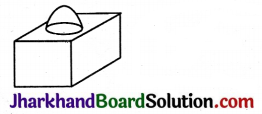Greatest diameter = 7 cm.
Surface area of the block = T.S.A. of the cube – Base area of the hemisphere + C.S.A. of the hemisphere
= 6 × 72 – πr2 + 2πr2
= 6 × 49 + πr2
= 6 × 49 + $$\frac{22}{7}$$ × 3.5 × 3.5
= 294 + 11 × 3.5
= 294 + 38.5
= 332.5 cm2.Question 5.
A hemispherical depression is cut out from one face of a cubical wooden block such that the diameter l of the hemisphere is equal to the edge of the cube. Determine the surface area of the remaining solid.
Solution: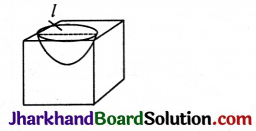Surface area of the remaining solid = Surface area of the cube + Surface area of the hemisphere
= 6l2 + 2πr2
= 6l2 + 2π$$\left(\frac{l}{2}\right)^2$$
= 6l2 + 2π$$\frac{l^2}{4}=\frac{l^2}{4}$$(24 + 2π) sq. units.

Question 6.
A medicine capsule is in the shape of a cylinder with two hemispheres stuck to each of its ends. The length of the entire capsule is 14 mm and the diameter of the capsule is 5 mm. Find its surface area.
Solution: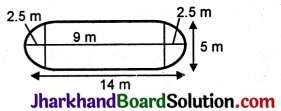Height of the cylindrical portion = 14 – 2.5 – 2.5 = 9m = h
r = 2.5 m.
Surface area of the capsule = Surface area of the cylindrical portion + Areas of the hemispherical regions
= 2πrh + 2πr2 + 2πr2
= 2πr(h + r + r)
= 2 × $$\frac{22}{7}$$ × 2.5 (9 + 2.5 + 2.5)
= 2 × $$\frac{22}{7}$$ × 2.5 (9 + 5)
=2× $$\frac{22}{7}$$ × 2.5 × 14
= 44 × 5
= 220 mm2.

Question 7.
A tent is in the shape of a cylinder surmounted by a conical top. If the height and diameter of the cylindrical part are 2.1 m and 4 m respectively, and the slant height of the top is 2.8 m, find the area of the canvas used for making the tent. Also, find the cost of the canvas of the tent at the rate of Rs. 500 per m2. (Note that the base of the tent will not be covered with canvas.)
Solution:
r = $$\frac{4}{2}$$ = 2m, h = 2.1, l = 2.8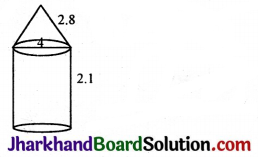Area of the canvas used = C.S.A. of the cylindrical portion + C.S.A. of the conical region
= 2πrh + πrl
= πr(2h + l)
= $$\frac{22}{7}$$ × 2(2 × 2.1 + 2.8)
= $$\frac{22}{7}$$ × 2(4.2 + 2.8)
= $$\frac{22}{7}$$ × 2 × 7
= 44 m2.
Cost of the canvas = Area × Rate
= 44 × 500
= Rs. 22000.Question 8.
From a solid cylinder whose height is 2.4 cm and diameter 1.4 cm, a conical cavity of the same height and same diameter is hollowed out. Find the total surface area of the remaining solid to the nearest cm2.
Solution:
Height of conical part = Height of cylindrical part h = 2.4 cm.
Diameter of cylindrical part = 1.4 cm, so, the radius of cylindrical part r = 0.7 cm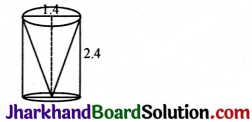Slant height of cylindrical part l = $$\sqrt{r^2+h^2}$$
= $$\sqrt{(0.7)^2+(2.4)^2}$$
= $$\sqrt{0.49+5.76}$$
= $$\sqrt{6.25}$$
= 2.5
The total surface area of the remaining solid = CSA of cylindrical part + CSA of conical part + Area of base of cylinder
= 2πrh + πrl + πr2
= 2 × $$\frac{22}{7}$$ × 0.7 × 2.4 + $$\frac{22}{7}$$ × 0.7 × 2.5 + $$\frac{22}{7}$$ × 0.7 × 0.7
= 4.4 × 2.4 + 2.2 × 2.5 + 2.2 × 0.7
= 10.56 + 5.50 + 1.56
= 17.60 cm2.

Question 9.
A wooden article was made by scooping out a hemisphere from each end of a solid cylinder, as shown in the figure. If the height of the cylinder is 10 cm, and its base is of radius 3.5 cm, find the total surface area of the article.
Solution: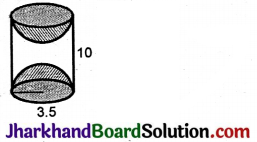Total surface area of the article = C.S.A. of the cylinder + Surface area of the hemisphere at the top + Surface area of the hemisphere at the bottom
= 2πrh + 2πr2 + 2πr2
= 2πr(h + r + r)
= 2 × $$\frac{22}{7}$$ × 3.5 (10 + 3.5 + 3.5)
= 2 × 22 × 0.5 × 17
= 22 × l × 17
= 374 cm2.